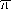Functions and CALL Routines

# ATAN2 Function

Returns the arc tangent of the ratio of two numeric variables.
 Category: Trigonometric

## Syntax

 ATAN2(argument-1, argument-2)

### Arguments

argument-1

specifies a numeric constant, variable, or expression.

argument-2

specifies a numeric constant, variable, or expression.

The ATAN2 function returns the arc tangent (inverse tangent) of two numeric variables. The result of this function is similar to the result of calculating the arc tangent of argument-1 / argument-2, except that the signs of both arguments are used to determine the quadrant of the result. ATAN2 returns the result in radians, which is a value between -and. If either of the arguments in ATAN2 is missing, then ATAN2 returns a missing value.

The ATAN2 function is similar to the ATAN function except that ATAN calculates the arc tangent of the angle from the value of one argument rather than from two arguments.

SAS statements Results
`a=atan2(-1, 0.5);`
`-1.107148718`
`b=atan2(6,8);`
`0.6435011088`
`c=atan2(5,-3);`
`2.1112158271`

 Functions:Previous Page | Next Page | Top of Page